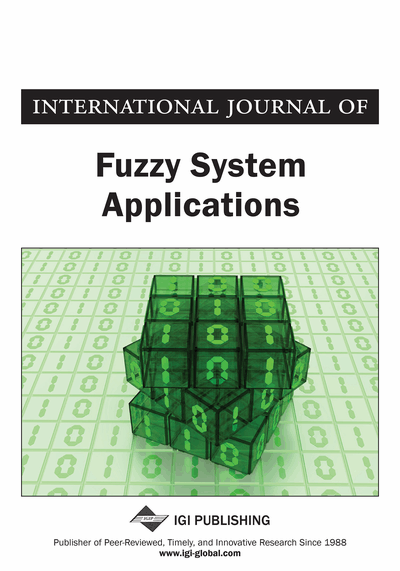# PSK Method for Solving Intuitionistic Fuzzy Solid Transportation Problems

P.Senthil Kumar
DOI: 10.4018/IJFSA.2018100104
OnDemand:
(Individual Articles)
Available
\$37.50
No Current Special Offers

## Abstract

This article proposes a method for solving intuitionistic fuzzy solid transportation problems (IFSTPs) in which only the transportation costs are represented in terms of intuitionistic fuzzy numbers (IFNs). The remaining parameters, namely: supply, demand and conveyance capacity, are all considered into crisp numbers. This type of STP is called a type-2 IFSTP. When solving the real life solid transportation problems (STPs) those tend to face the uncertainty state as well as hesitation due to many uncontrollable factors. To deal with uncertainty and hesitation many authors have suggested the intuitionistic fuzzy representation for the data. In this article, the author tried to categorise the STPs under the uncertain environment. He formulates the intuitionistic fuzzy STPs and utilizes the triangular intuitionistic fuzzy number (TIFN) to deal with uncertainty and hesitation. The PSK (P.Senthil Kumar) method for finding an intuitionistic fuzzy optimal solution for fully intuitionistic fuzzy transportation problem (FIFTP) is extended to solve the type-2 IFSTP and the optimal objective value of type-2 IFSTP is obtained in terms of TIFN. The main advantage of this method is that the optimal solution of type-2 IFSTP is obtained without using the basic feasible solution and the method of testing optimality. Moreover, the proposed method is computationally very simple and easy to understand. A case study is presented to illustrate the procedure of the proposed method.
Article Preview
Top

## 1. Introduction

The transportation problem is a special class of linear programming problem, widely used in the areas of inventory control, communication network, aggregate planning, employment scheduling, personal management and so on. In several real-life situations, there is a need for shipping the product from different origins (Factories) to different destinations (Warehouses). The transportation problem deals with shipping commodities from different origins to various destinations. The objective of the transportation problem is to determine the optimum amount of a commodity to be transported from various supply points (origins) to different demand points (destinations) so that the total transportation cost is minimum or total transportation profit is maximum. A minimization transportation problem involves cost data, in this case, the objective of solution is to minimize the total cost. On the other hand, a maximization transportation problem involves sales, revenue or profit data, in this case, the objective of solution is to maximize the total profit. The unit costs/profits, that is, the cost/profit of transporting one unit from a particular supply point to a particular demand point, the amounts available at the supply points and the amounts required at the demand points are the parameters of the transportation problem.

In the history of mathematics, Hitchcock (1941) originally developed a basic transportation problem. The transportation algorithm for solving transportation problems with equality constraints introduced by Dantzig (1963) is the simplex method specialized to the format of a table called transportation table. It involves two steps. First, we compute an initial basic feasible solution for the transportation problem and then, we test optimality and look at improving the basic feasible solution to the transportation problem. An Introduction to Operations Research Taha (2008) deals the transportation problem where all the parameters are crisp number. Xie et al. (2017) presented an upper bound on the minimal total cost of the transportation problem with varying demands and supplies.

The solid transportation problem is a generalization of the classical transportation problem in which three-dimensional properties are taken into account in the objective and constraint set instead of source (origin) and destination. Shell (1955) stated an extension of well-known transportation problem is called a solid transportation problem in which bounds are given on three items, namely, supply, demand and conveyance. In many industrial problems, a homogeneous product is transported from an origin to a destination by means of different modes of transport called conveyances, such as trucks, cargo flights, goods trains, ships and so on. Haley (1962) presented the solution procedure for solving solid transportation problem, which is an extension of the modified distribution method. Patel and Tripathy (1989) proposed a computationally superior method for a solid transportation problem with mixed constraints. Basu et al. (1994) developed an algorithm for finding the optimum solution of a solid fixed charge linear transportation problem.

For finding an optimal solution, the solid transportation problem requiresnon-negative values of the decision variables to start with a basic feasible solution. Jimenez and Verdegay (1996) investigated interval multiobjective solid transportation problem via genetic algorithms. Li et al. (1997a) designed a neural network approach for a multicriteria solid transportation problem. Efficient algorithms have been developed for solving transportation problems when the coefficient of the objective function, demand, supply and conveyance values are known precisely.

## Complete Article List

Search this Journal:
Reset
Volume 13: 1 Issue (2024)
Volume 12: 1 Issue (2023)
Volume 11: 4 Issues (2022)
Volume 10: 4 Issues (2021)
Volume 9: 4 Issues (2020)
Volume 8: 4 Issues (2019)
Volume 7: 4 Issues (2018)
Volume 6: 4 Issues (2017)
Volume 5: 4 Issues (2016)
Volume 4: 4 Issues (2015)
Volume 3: 4 Issues (2013)
Volume 2: 4 Issues (2012)
Volume 1: 4 Issues (2011)
View Complete Journal Contents Listing